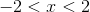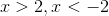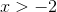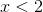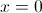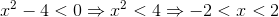# PSAT Math : Equations / Inequalities

## Example Questions

### Example Question #11 : Inequalities

What values of x make the following statement true?

|x – 3| < 9

–3 < x < 9

6 < x < 12

–6 < x < 12

x < 12

–12 < x < 6

–6 < x < 12

Explanation:

Solve the inequality by adding 3 to both sides to get x < 12.  Since it is absolute value, x – 3 > –9 must also be solved by adding 3 to both sides so: x > –6 so combined.

### Example Question #1 : How To Find The Solution To An Inequality With Addition

If –1 < w < 1, all of the following must also be greater than –1 and less than 1 EXCEPT for which choice?

|w|

w2

3w/2

w/2

|w|0.5

3w/2

Explanation:

3w/2 will become greater than 1 as soon as w is greater than two thirds. It will likewise become less than –1 as soon as w is less than negative two thirds. All the other options always return values between –1 and 1.

### Example Question #191 : Equations / Inequalities

Solve for.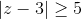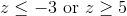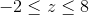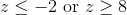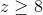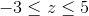Explanation:

Absolute value problems always have two sides: one positive and one negative.

First, take the problem as is and drop the absolute value signs for the positive side: z – 3 ≥ 5. When the original inequality is multiplied by –1 we get z – 3 ≤ –5.

Solve each inequality separately to get z ≤ –2 or z ≥ 8 (the inequality sign flips when multiplying or dividing by a negative number).

We can verify the solution by substituting in 0 for z to see if we get a true or false statement. Since –3 ≥ 5 is always false we know we want the two outside inequalities, rather than their intersection.

### Example Question #21 : Inequalities

If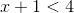and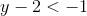, then what is the value of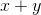?Explanation:

To solve this problem, add the two equations together: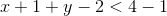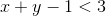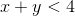The only answer choice that satisfies this equation is 0, because 0 is less than 4.

### Example Question #1 : How To Find The Solution To An Inequality With Addition

If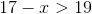, which of the following could be a value of?-Explanation:

In order to solve this inequality, you must isolateon one side of the equation.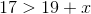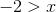Therefore, the only option that solves the inequality is### Example Question #22 : Inequalities

What values ofmake the statement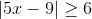true?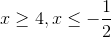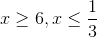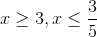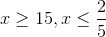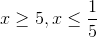Explanation:

First, solve the inequality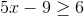: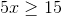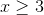Since we are dealing with absolute value,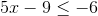must also be true; therefore: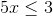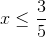### Example Question #1 : How To Find The Solution To An Inequality With Multiplication

If –1 < n < 1, all of the following could be true EXCEPT:

16n2 - 1 = 0

(n-1)2 > n

n2 < 2n

n2 < n

|n2 - 1| > 1

|n2 - 1| > 1

Explanation: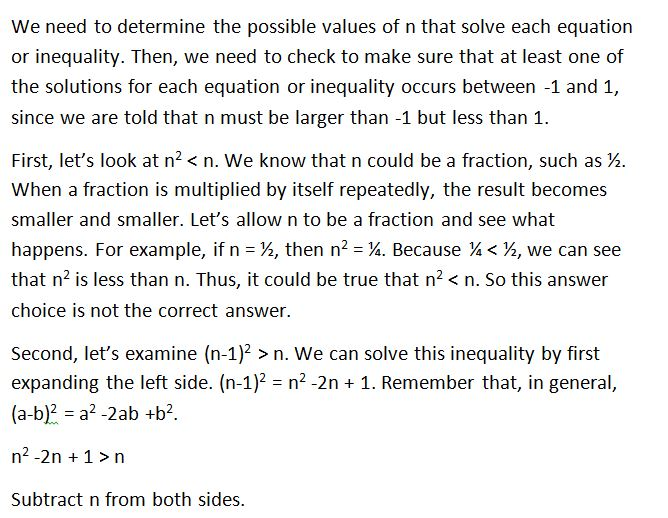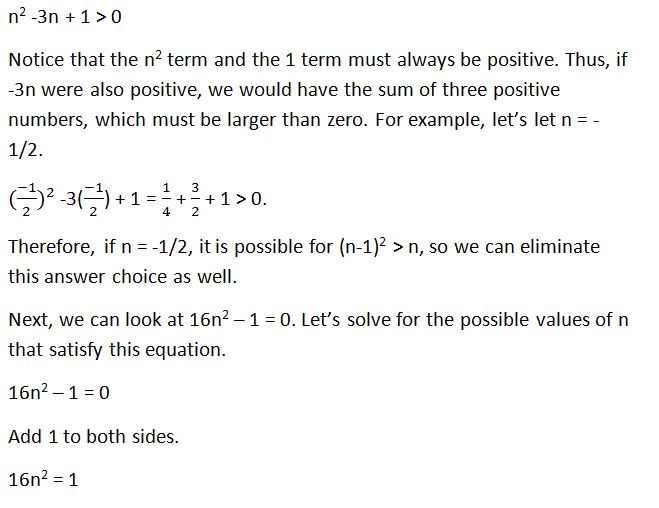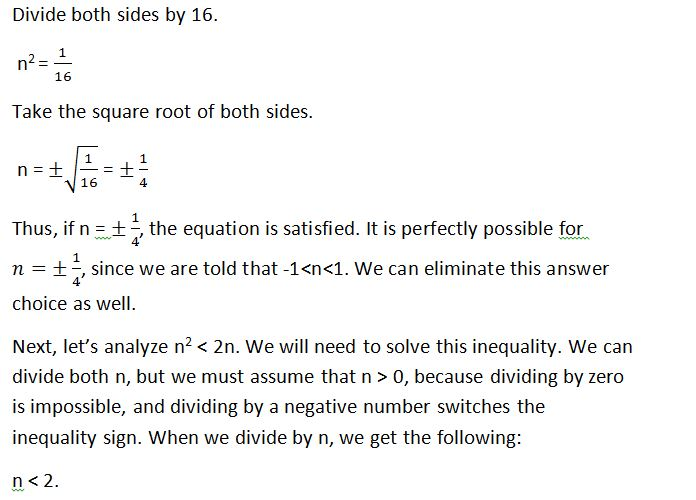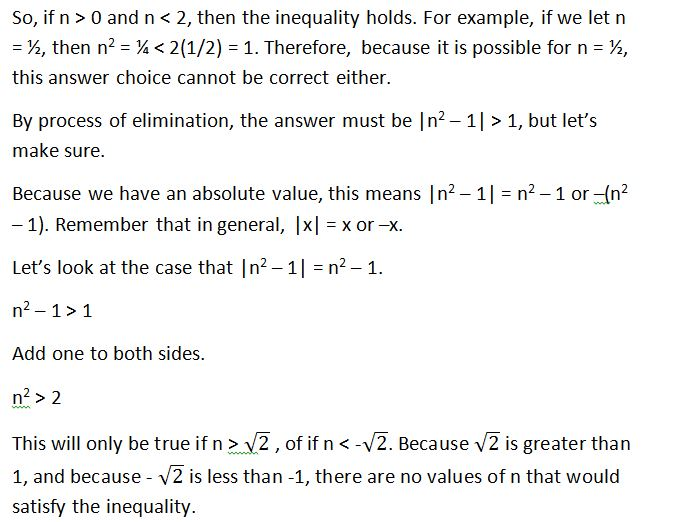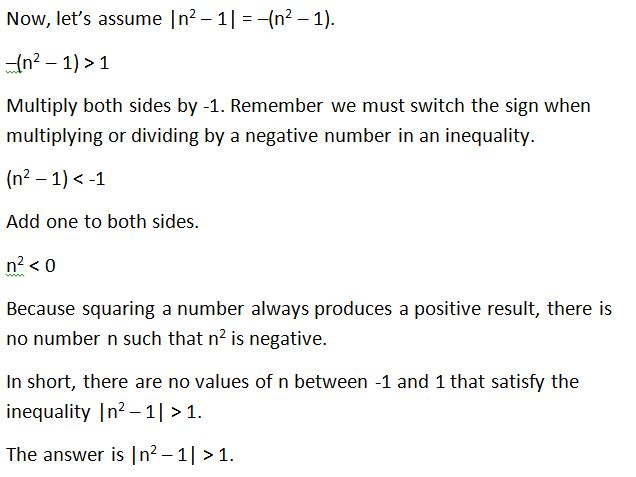### Example Question #11 : Inequalities

(√(8) / -x ) <  2. Which of the following values could be x?

-4

-3

All of the answers choices are valid.

-2

-1

-1

Explanation:

The equation simplifies to x > -1.41. -1 is the answer.

### Example Question #51 : New Sat Math Calculator

Solve for x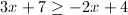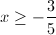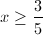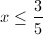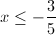Explanation: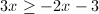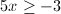### Example Question #5 : How To Find The Solution To An Inequality With Multiplication

We have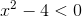, find the solution set for this inequality.